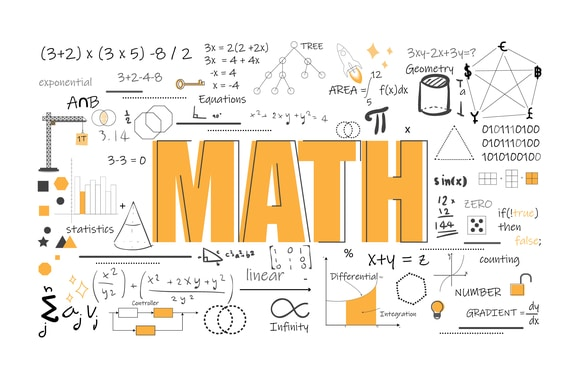# Simple Fractional Equations

Welcome to Class !!

In today’s Mathematics class, We will be looking at Simple Fractional Equations. We hope you enjoy the class!##### SOLVING EQUATION EXPRESSIONS

WORD PROBLEMS

Worked Examples:

1. Find 1/4 of the positive difference between 29 & 11
2. The product of a certain number and 5 is equal to twice the number subtracted from 20. Find the number
3. The sum of 35 and a certain number is divided by 4 the result is equal to double the number. Find the number.

Solutions:

1. Positive Difference 29 – 11 = 18



1. Let the number be x

x X 5 = 20 – 2x

5x = 20 – 2x

5x + 2x = 20

7x = 20

x = 20/7 = 2

1. Let the number be n

sum of 35 and n = n + 35

EVALUATION

1. From 50 subtract the sum of 3 & 5 then divide the result by 6
2. The sum of 8 and a certain number is equal to the product of the number and 3 find the number

###### SOLVING EQUATION EXPRESSIONS WITH FRACTION

Always clear fractions before beginning to solve an equation: –

To clear fractions, multiply each term in the equation by the LCM of the denominations of the fractions.

Examples:

Solve the following

2x + 18 + 10 + 5x = 0

1. 2x =

Multiply by the LCM (14)

28x = 31x – 33

28x – 31x = -33

-3x = -33

x = 33/3 = 11

EVALUATION

Solve the following equations.

##### Furthermore, we can consider the word equations or expressions into:
• Sum & Differences
• Products
• Expressions with fractions & equations

SUM & DIFFERENCES

The sum of a set of numbers is a result obtained when the numbers are added together. The difference between two numbers is a result of subtracting one number from the other.

Worked Examples:

1. Find the sum of -2 & -3.4
2. Find the positive difference between 19 & 8
3. The difference between two numbers is 7. If the smaller number is 7 find the other.
4. The difference between -3 and a number is 8, find the two possible values for the number.
5. Find the three consecutive numbers whose sum is 63.

Solutions:

1. -2 + -3.4 = -5.4
2. 19 – 8 = +11
3. let the number be Y i.e. Y -7 = 7

i.e. Y = 7 + 7 = 14

1. Let M represent the number

M – (-3) = 8

m + 3 = 8

m = 8 – 3

m  = +5

also -3 – m = 8

-m = 8 + 3

-m = 11

m = -11

the possible values are +5 & -11

1. Consecutive numbers are 1, 2, 3,4,5,6, Consecutive odd numbers are

1, 3,5,7,9……….. consecutive even numbers are 2, 4, 6, 8,10……….

Representing in terms of X, we have 2X, 2X + 2, 2X + 4, 2X + 6, 2X + 8, 2X + 10…………

for consecutive even numbers, we have X, X + 2, X + 4, X + 6…….

for consecutive odd numbers, we have  X + 1, X + 2, X + 3, X + 4…

for consecutive numbers.

let the first number be x,

let the second number be x + 1

let the third number be x + 2

Therefore x + x + 1 + x + 2 = 63

3x + 3 = 63

3x = 63 – 3

3x = 60

x = 60 /3

= 20

The numbers are 20, 21, and 22.

EVALUATION

1. Find the sum of all odd numbers between 10 and 20
2. The sum of four consecutive odd numbers is 80 find the numbers
3. The difference between 2 numbers is 9, the largest number is 32 find the numbers.

PRODUCTS

The product of two or more numbers is the result obtained when the numbers are multiplied together.

Worked Examples:

1. Find the product of – 6, 0.7, &
2. The product of two numbers is 8. If one of the numbers is 1/4 find the other.
3. Find the product of the sum of -2 & 9 and the difference between -8 & -5.

Solutions

1. Products -6 x 0.7 x 20/3

-6 x 7/10 x 20/3 =

= -2 x 7 x 2 = -28

1. Let the number be x

$\frac{1}{4}$ X x = 8 multiply both sides by 4

x = 8 x 4 = 33

1. Sum = -2 + 9 = 7

Difference = -5-(-8) = -5 + 8 = 3

Products= 7 x 3 = 21

EVALUATION

1. The product of three numbers is 0.084 if two numbers are 0.7 & 0.2 find the third number
2. Find the product of the difference between 2 & 7 and the sum of 2 & 7
3. From 50 subtract the sum of 3 & 5 then divide the result by 6
4. The sum of 8 and a certain number is equal to the product of the number and 3 find the number

We have come to the end of this class. We do hope you enjoyed the class?

Should you have any further question, feel free to ask in the comment section below and trust us to respond as soon as possible.

In our next class, we will be talking about Change Of Subject Formulae. We are very much eager to meet you there.

For more class notes, videos, homework help etc download our Mobile App HERE

Join ClassNotes.ng Telegram Community HERE for exclusive content and support

Don`t copy text!